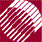ECE532 Biomedical Optics © 1998 Steven L. Jacques, Scott A. Prahl Oregon Graduate Institute

Terminate a photon by roulette

The decision on whether or not to terminate a photon is made using the roulette method. If the photon weight (W) becomes less than the value THRESHOLD chosen to be 0.01 (see header), then the photon survival is decided by roulette. A random number is drawn and if that random number is less than CHANCE (chosen to equal 0.10) then the photon is terminated. Otherwise, the current photon weight is increased by the factor 1/CHANCE (or 10-fold) and the photon continues to propagate. This method allows 9 out of 10 photons to be terminated, but the one surviving photon is given added weight so as to properly conserve total photon energy. A photon is terminated by letting photon_status = DEAD. Then the launch DO-WHILE loop will recognize the photon is terminated and launch a new photon.

/**** CHECK ROULETTE
If photon weight below THRESHOLD, then terminate photon using Roulette technique.
Photon has CHANCE probability of having its weight increased by factor of 1/CHANCE,
and 1-CHANCE probability of terminating.
*****/
if (W < THRESHOLD) {
if (RandomNum <= CHANCE)
W /= CHANCE;